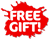ISBN: 978-1-988586-02-1

## Level 2 Algebra 2.6 Learning Workbook (new edition)Phyl Haydock

• 895

Level 2 Algebra Learning Workbook covers NCEA Level 2 Achievement Standard 91261 (Mathematics and Statistics 2.6): Apply algebraic methods in solving problems.

This standard is externally assessed and worth 4 credits.

The workbook features:

• bite-sized sections of notes with student-friendly explanations
• worked examples to illustrate fundamental concepts
• plenty of exercises so students can improve their skills and practise problem solving
• answers to all exercises, which are provided at the end of the book
• a practice assessment task.

An index gives easy access to important terms and definitions highlighted in the text.
This workbook covers algebraic expressions and equations at curriculum level 7, and their use in solving problems. This includes rational, exponential and logarithmic expressions, and the forming and solving of exponential and quadratic equations. Also covered is the use of the discriminant in finding the nature of the roots of quadratic equations.

The concise notes and write-on exercises keep student work together in a compact format, providing a useful end-of-topic revision tool.

This workbook is ideal for use in the classroom or for homework, home study and exam revision.

Published: 12/2019